## Kalman filter for simple average belt flow measurement

This board is to show, discuss and archive useful combinator- and logic-creations.
Smart triggering, counters and sensors, useful circuitry, switching as an art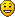, computers.
mm.lion
Burner Inserter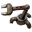Posts: 16
Joined: Mon Dec 31, 2018 11:39 am
Contact:

### Kalman filter for simple average belt flow measurement

I'd like to share a very compact design to measure a belt flow. I know that there is a way to measure the flow by connecting 100 belts in a line together, but it is too heavy in my opinion. Here is another simple solution.

On the picture 225k and 112k means that the average flow is equal to 22.5 and 11.2 items per second.The main idea is to use the simplified Kalman filter: if f_n denotes the instant flow speed at tick n, then prediction of the average flow is

Code: Select all

``K_(n+1)  =  a * f_n  +  (1-a) * K_n``
where a is a constant between 0 and 1, which controls duration of the filter's reaction to the changes. For example, if representative measurement time is 3 sec = 180 tick, and computation takes 2 ticks, then a=1/90.

The instant flow speed f_n is equal to the number I_n of items on a belt multiplied by 45/8 (for express belts). I also decided to multiply I_n by 10000 due to integer computations in factorio. So

Code: Select all

``````	F_(n+1) = (  (10000*45/8) * I_n + 89 * F_n ) / 90 = ( 56250 * I_n + 89  * F_n) / 90
``````
Unfortunately, integer computations gives a small error at the end (for example, a fully compressed belt leads to the value 449k instead of 450k), so it is better to rise 56250 a little and have a nice round numbers like 450k. So, I used the following formulae for normal/fast/express belts:

Code: Select all

``````	F_(n+1) = ( 18800 * I_n + 89  * F_n) / 90         (normal)
F_(n+1) = ( 37500 * I_n + 89  * F_n) / 90         (fast)
F_(n+1) = ( 56300 * I_n + 89  * F_n) / 90         (express)
``````
If prolonged duration of filter's reaction is needed, numbers (89,90) can be substituted by (299,300) (for 10 seconds representative reaction time), or any other pair (N-1,N).

I'm interested if this design seems interesting/usable.

quyxkh
Filter Inserter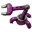Posts: 964
Joined: Sun May 08, 2016 9:01 am
Contact:

### Re: Kalman filter for simple average belt flow measurement

I use that to maintain adaptive sushi belts. I never knew it by that name, just as "exponential-decay average". I use read-pulse metering, though, since that works for any belt speed and doesn't get confused if the belt backs up.

edit: here's mine, I'm not so interested in getting an exact rate value but I do want to be able to fine-tune the supply, that's a ... did you say twice? twenty-second-decay averager so I can keep very-low-volume supplies on the belt. The extra combinator eventually drains missing-item counts to zero.

FuryoftheStars
Smart Inserter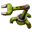Posts: 1207
Joined: Tue Apr 25, 2017 2:01 pm
Contact:

### Re: Kalman filter for simple average belt flow measurement

Thank you both for sharing these. At the moment, I use a regular counter + clock to achieve per time interval throughputs. If I want per sec average, I then have to feed the output through an extra arithmetic combinator before the display. Uses somewhere in the neighborhood of 8-10 combinators. I've always wanted something smaller and that gave closer to "live" throughput readings.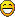mm.lion
Burner InserterPosts: 16
Joined: Mon Dec 31, 2018 11:39 am
Contact:

### Re: Kalman filter for simple average belt flow measurement

quyxkh wrote:
Tue Jan 11, 2022 6:27 pm
I use that to maintain adaptive sushi belts. I never knew it by that name, just as "exponential-decay average". I use read-pulse metering, though, since that works for any belt speed and doesn't get confused if the belt backs up.
Yes, your are right, pulse measuring is obviously better. I play a little with your idea to get it work using only 3 combinators.

It turns out that it is doable, but all combinators should be set to the same magic number 2*123=246 to work!Are there any other magic factorio numbers?

PS It seems to me that throughput of the whole main bus can be measured by using only these three combinators. You just connect different belts to the same input of the left combinator...farcast
Long Handed Inserter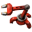Posts: 60
Joined: Fri Jul 06, 2018 8:25 am
Contact:

### Re: Kalman filter for simple average belt flow measurement

mm.lion wrote:
Thu Jan 13, 2022 4:39 pm
It turns out that it is doable, but all combinators should be set to the same magic number 2*123=246 to work!Are there any other magic factorio numbers?
The magic number comes from how the accumulated value = expected items per tick * input multiplier * |divisor|. You're expecting 22.5 items per second, or 0.375 items per tick. You want the accumulated value to be 22.5 * 1000, so input multiplier * |divisor| = 22,500 / 0.375 = 60,000 , or approximately 246^2 (closer to 245^2).

If you use the output of the divider as the average, then the divisor will have no effect on the final output value and the input multiplier can be interpreted as a time unit conversion. Want the output to be items per second? Multiply by 60 ticks per second. Items per minute? 3600 ticks per minute. Just remember that the input multiplier needs to be negative if you want the output to have the same sign as the input since the divisor must be negative.
Efficient inefficient design.

disentius
Filter InserterPosts: 659
Joined: Fri May 12, 2017 3:17 pm
Contact:

### Re: Kalman filter for simple average belt flow measurement

I got this one (exponential average) a while ago from this forum. it is very accurate when you set the S higher, or use 2 in a row.
input top left, set the multiplier to the desired number for the resolution, output top right, fading factor CC bottom right

mm.lion
Burner InserterPosts: 16
Joined: Mon Dec 31, 2018 11:39 am
Contact:

### Re: Kalman filter for simple average belt flow measurement

farcast wrote:
Thu Jan 13, 2022 6:09 pm
mm.lion wrote:
Thu Jan 13, 2022 4:39 pm
It turns out that it is doable, but all combinators should be set to the same magic number 2*123=246 to work!Are there any other magic factorio numbers?
The magic number comes from how the accumulated value = expected items per tick * input multiplier * |divisor|. You're expecting 22.5 items per second, or 0.375 items per tick. You want the accumulated value to be 22.5 * 1000, so input multiplier * |divisor| = 22,500 / 0.375 = 60,000 , or approximately 246^2 (closer to 245^2).

If you use the output of the divider as the average, then the divisor will have no effect on the final output value and the input multiplier can be interpreted as a time unit conversion. Want the output to be items per second? Multiply by 60 ticks per second. Items per minute? 3600 ticks per minute. Just remember that the input multiplier needs to be negative if you want the output to have the same sign as the input since the divisor must be negative.
Yes, i know the math, it computed 246 for a smileThis design actually works for any throughput not only 22.5, since 1 pulse = 60 items/sec, so it remains to put a=sqrt(60 000)=244.9 in exponential average to make all numbers equal. I think it is really nice to have 246,246,246disentius wrote:
Thu Jan 13, 2022 7:00 pm
I got this one (exponential average) a while ago from this forum. it is very accurate when you set the S higher, or use 2 in a row.
input top left, set the multiplier to the desired number for the resolution, output top right, fading factor CC bottom right
Thanks!

quyxkh
Filter InserterPosts: 964
Joined: Sun May 08, 2016 9:01 am
Contact:

### Re: Kalman filter for simple average belt flow measurement

Yeah, the extra drain is only useful for extremely-low-volume flow metering, I could probably lose it if I'd just up the premuiltiplier to 1'000'000 from 1'000. I like those two because they give three digits of precision on the mouseover readout, this setup reads -75k, -150k, -225k for instance:
pic+bp
and with flow rates this high the drain combinator is just kinda there, it's only when you want to meter flows down to around 4/min that it helps.

mmmPI
Smart InserterPosts: 1601
Joined: Mon Jun 20, 2016 6:10 pm
Contact:

### Re: Kalman filter for simple average belt flow measurement

Thanks you for taking the time to add the explanations it is interesting to me therefore i should try to use it somewhere at least once